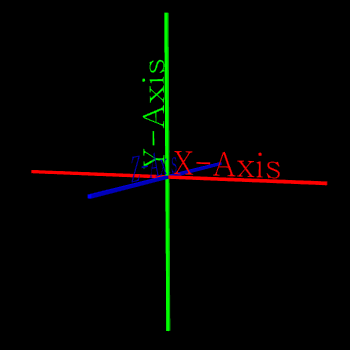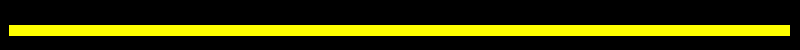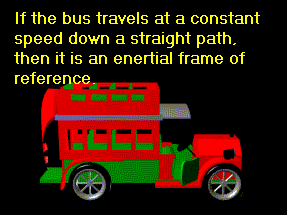# Inertial Frame of Reference

An inertial frame of reference has a constant velocity. That is, it is moving at a constant speed in a straight line, or it is standing still. Understand that when something is standing still, it has a constant velocity. Its velocity is constantly zero meters per second.

In the animation below an (x, y, z) axes represents an inertial frame of reference. This set of axes is moving left to right at a constant velocity.

• Inertial Frame of Reference
• (X, Y, Z) Axes with Constant Velocity
• No AccelerationTo say that the velocity of a frame of reference is constant is the same as saying that the frame is not accelerating. So, we could define an inertial frame of reference to be a coordinate system which is not accelerating.

Such a constant velocity frame of reference is called an inertial frame because the law of inertia holds in it. That is, an object whose position is judged from this frame will tend to resist changes in its velocity; it obeys the law of inertia. An object viewed with this frame will not spontaneously change its velocity. An object within this frame will only change its velocity if an actual non-zero net force is applied to it.

There are several ways to describe an inertial frame. Here are a few descriptions:

• An inertial frame of reference is a frame of reference with constant velocity.
• An inertial frame of reference is a non-accelerating frame of reference.
• An inertial frame of reference is a frame of reference in which the law of inertia holds.
• An inertial frame of reference is a frame of reference in which Newton's laws of motion hold.
• In an inertial frame of reference no fictitious forces arise.

Consider the act of juggling, a definite demonstration of Newton's laws of motion. It is just as easy (or just as difficult) to juggle balls in a room which is standing still as it is to juggle in a bus which is traveling smoothly down a straight road at constant speed. (Disregard problems with elbow room on the bus, or imagine one with no seats in it.) In fact, the juggler on the bus could not determine that the bus was moving based on any clues gathered from the motion of the balls. They would move through the air within the moving bus exactly as if they were being tossed about within the still room - as long as the bus traveled smoothly down a straight road at constant speed; that is, as long as the bus moved with a constant velocity.The physics of typical mechanics is always the same when it is done within a constant velocity frame of reference. Without visual aids, such as viewing the scenery going by, and without sound clues, such as the noise of the engine and drive train, the juggler physicist on the constant velocity bus could perform no experiment to determine if the bus was moving or was parked. Such frames of reference as our constant velocity bus are called inertial frames of reference.

The bus would cease to be an inertial frame of reference while it changed its velocity. That would happen if it slowed down, or if it sped up, or if it turned around a corner. Each of these changes in velocity would constitute an acceleration. And, while the bus was accelerating, the act of juggling could get quite difficult. For example, if the bus driver slammed on the brakes while some of the balls were in flight, those balls would seem to fly forward from the juggler's perspective, assuming that the juggler was facing the front of the bus. From the viewpoint of the juggler it would seem as if some unknown force had pushed the balls away from her, making them fly up toward the front of the bus. The juggler, too, would feel a push toward the front of the bus. But remember, in this situation the bus is no longer an inertial frame of reference. Its velocity is changing; it is now an accelerating frame of reference. So, the law of inertia and Newton's laws of motion no longer hold. This accelerating frame is called a non-inertial frame of reference.